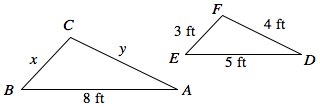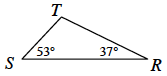### Home > CC2MN > Chapter 4 > Lesson 4.4.2 > Problem4-140

4-140.$ΔABC$ and $ΔDEF$ are similar.

Find the scale factor between the two corresponding sides that are given and utilize it with the respective side lengths from  $\triangle DEF$ to get $x$ and $y$.

The scale factor is $\frac{8}{5}$.

1. Find $x$.

$x=\frac{24}{5}$

2. Find $y$.

$y=\frac{32}{5}$

3. Find the ratio of the perimeters of the two triangles.

Find the perimeters of both triangles and compare them as a ratio. What do you notice?

Perimeter of $\triangle ABC$: $19.2$ ft
Perimeter of $\triangle DEF$$12$ ft

4. Can you predict the ratio of the areas of the two triangles? If so, state it. If not, write the information you would need or the question you would need to answer first.

According to the Math Notes box from Lesson 4.4.2:

The area ratio is $\left(\frac{\text{new area}}{\text{original area}}\right)=\left(\text{scale factor}\right)^2$.

Try predicting the area ratio of the two triangles. What do you get?

$\left( \frac{8}{5} \right)^2 = \frac{64}{25}$

5.Triangle $RST$ is congruent to triangle $DEF$. Based on this, what are the side lengths of triangle $RST$? What is the measure of angle $E$?

Examine the two triangles and the corresponding sides or angles to determine each measure.